Author Message
Skit3000Joined: 11 May 2002
Posts: 2166
Location: The NetherlandsPosted: Fri Apr 01, 2005 7:18 am    Post subject: How to avoid a lot of @equals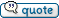If you have a word and you want to check if it's value is "A", "B", "C", "D" or "M", you normally need a lot of @equal functions to do this. It makes your code unreadable and it will become quite long.

To avoid this problem, you can use the @pos function to check if the word is in the string "|A|B|C|D|M|". If so, the @pos function will return a value greater than zero. If you like, you can combine the @pos function with the "EXACT" parameter.

The first example shows how such a "A" or "B" or "C" statement looks like:

 Code: %%word = duck if @equal(%%word, cow) @equal(%%word, chicken) @equal(%%word, duck) @equal(%%word, pig) @equal(%%word, bird) @equal(%%word, sheep) @equal(%%word, cat) @equal(%%word, dog) @equal(%%word, ape)   info Done end

The second shows how you can reduce all above code to some smaller one:

 Code: %%word = duck if @greater(@pos(|%%word|,|cow|chicken|duck|pig|bird|sheep|cat|dog|ape|),0)   info Done end

_________________
[ Add autocomplete functionality to your VDS IDE windows! ]
Voor Nederlandse beginners met VDS: bekijk ook eens deze tutorial!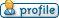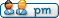Skit3000Joined: 11 May 2002
Posts: 2166
Location: The NetherlandsPosted: Fri Apr 01, 2005 7:25 am    Post subject:Another tip on how to avoid a lot of @both functions if you want to check if the first @equal is true, the second one is true, etc:

 Code: rem The "normal" way if @both(@both(@both(@both(@both(@both(@both(@equal(1,1),@equal(2,2)),@equal(3,3)),@equal(4,4)),@equal(5,5)),@equal(6,6)),@equal(7,7)),@equal(8,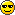)   info Done end

 Code: rem This way, the results of the @equals are examined as a string, so you don't need a lot of @bothsif @equal(@equal(1,1)@equal(2,2)@equal(3,3)@equal(4,4)@equal(5,5)@equal(6,6)@equal(7,7)@equal(8,,11111111)   info Done end

If you want not all the @equals to be true, but some of them (for example 2) may be false, you can change the "11111111" into "111111"..._________________
[ Add autocomplete functionality to your VDS IDE windows! ]
Voor Nederlandse beginners met VDS: bekijk ook eens deze tutorial!Skit3000Joined: 11 May 2002
Posts: 2166
Location: The NetherlandsPosted: Fri Apr 01, 2005 7:34 am    Post subject:Here is an example which combines the two methods shown above. It check if "A is A" and "B is not C", etc., without having to do a lot of @boths...Code: rem Old method if @both(@both(@both(@both(@equal(Jack,Jack),@not(@equal(duck,cow))),@equal(1,1)),@not(@equal(sheep,dog))),@not(@equal(text,book)))   info Done end

 Code: rem New method, use || for false and |1| for true: if @equal(|@equal(Jack,Jack)|@equal(duck,cow)|@equal(1,1)|@equal(sheep,dog)|@equal(text,book)|, "|1||1|||")   info Done end

_________________
[ Add autocomplete functionality to your VDS IDE windows! ]
Voor Nederlandse beginners met VDS: bekijk ook eens deze tutorial!Serge
Professional Member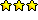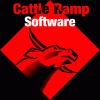Joined: 04 Mar 2002
Posts: 1480
Location: AustraliaPosted: Sat Apr 02, 2005 1:11 am    Post subject:excellent work there skitserge_________________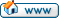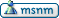FreezingFireJoined: 23 Jun 2002
Posts: 3508Posted: Sun Apr 03, 2005 9:39 pm    Post subject:Nice job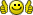_________________FreezingFire VDSWORLD.com Site Admin TeamDisplay posts from previous: All Posts1 Day7 Days2 Weeks1 Month3 Months6 Months1 Year Oldest FirstNewest First
 All times are GMT Page 1 of 1